# Power Content In Multiple-Tone AM

## Power Content In Multiple-Tone AM

A multiple-tone amplitude modulation is that type of modulation in which the modulating signal consists of more than one frequency components.

### Mathematical Expression

Let us consider that a carrier signal A cos ωct is modulated by a baseband or modulating signal x(t) which is expressed as :

As we know, the general expression for AM wave is :

Now, putting the value of x(t) from equation (1) in equation (2), we get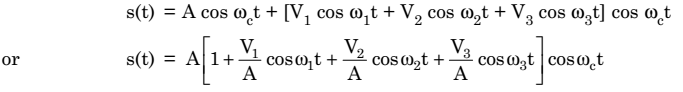……….. (3)

But we know that,Therefore,………………. (4)

Where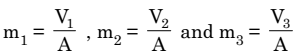are  the modulation indexes of the corresponding frequency components.

The expression for AM wave in equation (4) may further be expanded as :…… (5)

Now we know that the total power in AM is given as

P= carrier power + sideband power

Pt = P + P ……….. (6)

The carrier power Pc is given as :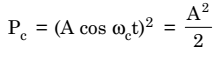……………. (7)

Similarly, the sideband power is given as :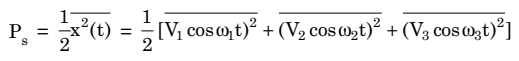……………. (8)

Putting the values of V1, V2 and V3 in equation (8), we get,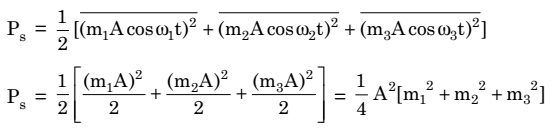…………. (9)

Now putting the value of Pand Ps in equation (6), we get: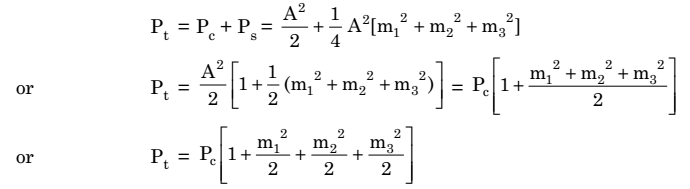This expression may be extended upto to n-modulating terms i.e.,…………… (10)